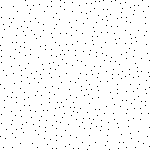# Question about procedural forest/grass generation

Hello,

I have been looking into this topic for quite some time now and can’t really find a solotion other then randomly placing objects in a specified area using Random.Range…

What I would like to do is generate plots for trees and grass to spawn using something like peril noise but I can’t figure it out. Can someone just simply explain to me how you would generate a forest using something like peril noise similar to this example:Were all the dots are trees.

Current system I have just generates trees way to far apart and makes the world seem bare:This script generates a forest based on some noise texture.
The noise texture can be though of as a “likelyness” map describing what the change of a tree spawning is at a given position. This can be controlled with the density variable. With a density of 1 the script produces this result:

The script picks a random size based on two values. This can of course be changed as well as doing the same for rotation.

``````using UnityEngine;

public class ForestGenerator : MonoBehaviour {

public GameObject tree;
public float minTreeSize;
public float maxTreeSize;
public Texture2D noiseImage;
public float forestSize;
public float treeDensity;

private float baseDensity = 5.0f;

// Use this for initialization
void Start () {
Generate();
}

public void Generate() {

for(int y = 0; y < forestSize; y++) {

for(int x = 0; x < forestSize; x++) {

float chance = noiseImage.GetPixel(x, y).r / (baseDensity/treeDensity);

if (ShouldPlaceTree(chance)) {
float size = Random.Range(minTreeSize, maxTreeSize);

GameObject newTree = Instantiate(tree);
newTree.transform.localScale = Vector3.one * size;
newTree.transform.position = new Vector3(x, 0, y);
newTree.transform.parent = transform;
}
}
}
}

//Returns true or false given some chance from 0 to 1
public bool ShouldPlaceTree(float chance) {
if (Random.Range(0.0f, 1.0f) <= chance) {
return true;
}
return false;
}
}
``````

Hope it helps as inspiration